# Electronics and Communication Engineering - Exam Questions Papers

1.

Px(X) = M exp(- 2 |x|) + N exp(- 3 |x|) is the probability density function for the real random variable X, over the entire x axis. M and N are both positive real numbers. The equation relating M and N is

 A.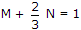B.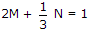C. M + N = 1 D. M + N = 3

Explanation:

Using property of probability density ofPxdx = 1[M exp(- 2 |x|) + N exp(- 3|x|)]dx

1 ⇒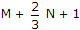.

2.

The electric field component of a time harmonic plane EM wave travelling in a non magnetic lossless dielectric medium has an amplitude of 1 V/m. If the relative permittivity of the medium is 4, the magnitude of the time-average power density vector (in W/m2) is

 A.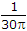B.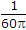C.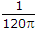D.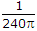Explanation: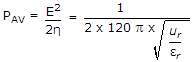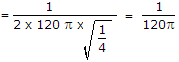3.

The open loop transfer function of a unity feedback control system is given as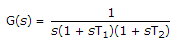The phase cross over frequency and the gain margin are respectively.

 A.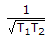and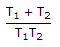B.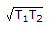andC.and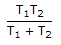D.andExplanation:

At phase cross over frequency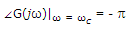-90° - tan- 1 ωT1 - tan- 1 ωcT2 = - p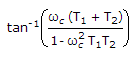= 90°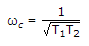GM = 1/a where a = | G()|ω=ωc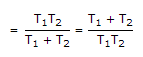.

4.

A unity negative feedback closed loop system has a plant with the transfer function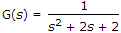and a controller Gc(S) in the feed forward path. For a unit set input, the transfer function of the controller that gives minimum steady state error is

 A.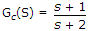B.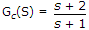C.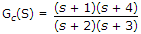D.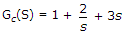Explanation: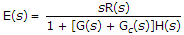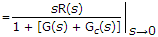so, Gc(s)|s → 0 should be maximum for low error = ∞

Gc(s)|s → 0.

5.

Find current i.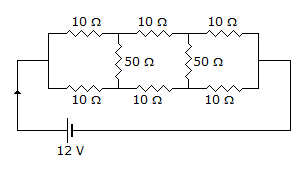A. 4/5 A B. 5/4 A C. 1.23 A D. 1.32 A

Explanation:

The given circuit can be compared with Wheatstone's Bridge

Req = (10 + 10 + 10) || (10 + 10 + 10) = 30 || 30 = 15 Ω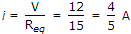.